#### Quantum Statistics, Physics tutorial

Introduction:

Quantum Mechanic Fermi-Dirac statistics are developed to compute the occupancy of the states. Special attention is provided to analytic approximations of the Fermi-Dirac integral and to its approximate conditions in the non-degenerate and the lightly degenerate regime.

Quantum statistics takes into account two outcomes of quantum mechanics, namely (a) The Pauli Exclusion Principle that limits the number of electrons occupying a state of energy E and (b) The finiteness of the number of states in an energy  interval E and E + dE . The finiteness of states is an outcome of the Schrodinger equation. The Quantum statistics assist us to correct all the inadequacies of the classical theory such as (a) Incapability to correctly deal by indistinguishable particles led us to Gibbs paradox and this can be corrected through resorting to a purely ad-hoc device of dividing the thermodynamic probability by N! (b) Problem of black body radiation can't be handled in the domain of classical process however max plank propose a remarkable idea for the resolution of the problem through deriving the Planck's law of black body radiation.

Towards Quantum Statistics:

In the classical physics we hypothesize that it is possible to find out the position and momentum coordinates of a gaseous molecule/atom concurrently as accurately as we like. All that we have to do is to follow its trajectory as it moves in the space. This signifies that these particles are distinguishable and can be labeled, however this is not true.

You will remember that the Heisenberg's un-certainly principle forbids determination of the position. Whenever the uncertainties in the measurements of q and p are Δq and Δp, correspondingly, we have:

Δq Δp ≥ h/4π

Here (h = 6.63 x 10-34 Js) is Planck's constant. That is, the product Δq Δp can't be made less than h/4π. Therefore it doesn't make much sense to talk regarding the trajectory of a particle. Furthermore, the task of labeling particles is just impossible and whenever we study the behavior of an assembly of similar particles statistically, we must treat it as a collection of indistinguishable particles.

Lord Kelvin spoke regarding two dark clouds on the horizon of classical physics, the heat capacity of solids and the black body radiation and this shook the structure of classical physics to its very foundations.

Heat capacity of solids:

You would remember that the solids behave as a collection of independent harmonic oscillators, and energy related with them is equivalent to 3NAKBT, here NA is Avogadro's number. Therefore, heat capacity at constant volume is constant, equivalent to 3R in spite of the substance.

CV = (∂u/∂T)V = 3R = 24.9 J mol-1 K1

The famous Dulang and Petit's law doesn't represent what experiments reveal regarding temperature variation of the heat capacity.

The deviations from this law, specifically in the low temperature area are striking, as represented in the figure shown below.

As 'T' reduces beneath the room temperature, CV as well reduces and becomes zero at absolute zero.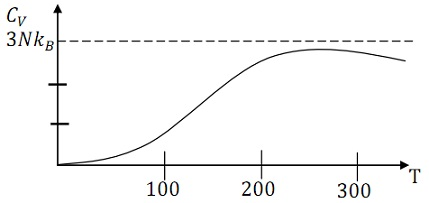A qualitative theoretical description was given by Einstein, by using Planck's idea on the quantization of energy.

The key to Einstein's success was that he rejected the law of equipartition of energy. The mean energy of the classical oscillator is represented by E‾ = KBT.

In the quantum theory, we have: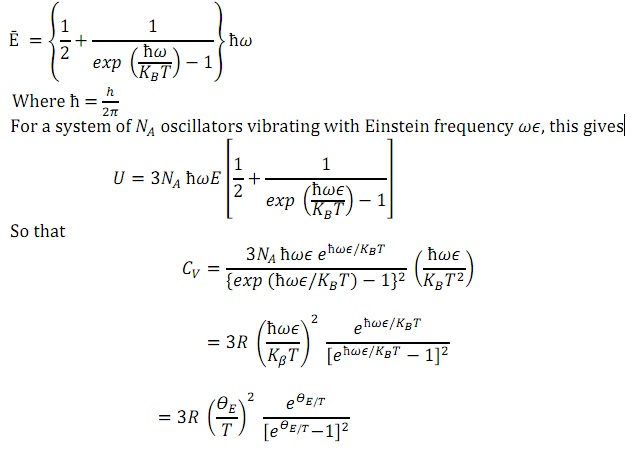Debye (1912) afterward refined Einstein's theory and obtained an excellent agreement by means of experiments.

The heat capacities of metals as well pose an interesting puzzle, however a challenge, to the classical physicists. You are familiar that each and every metal includes free electrons. If we suppose that such electrons comprise a monoatomic gas, they must contribute an amount (3/2) R to CV. Therefore, the heat capacity of a metal must be 3R + (3/2) R = (9/2) R. Though, we experimentally find out that the metal obey the Dulong-Petit's law as good as do insulators.

The Problem of Black Body Radiation:

We now take the common place phenomenon of black body radiation. It deserves an exclusive place in physics because it gave birth to the quantum theory.

If a body is heated, it emits electromagnetic waves (that is, from its surface) in all directions over a wide range of frequencies.  The spectrum of radiated frequencies from 0 to ω peaks at a frequency that is proportional to the absolute temperature of the body. Assume that such thermal radiation is contained in side a hollow cavity whose walls are opaque to radiation and maintained at a constant temperature.

The radiation in the interior should, thus have precisely the similar spectral distribution as that of black body radiation. In another word, the energy distribution over different wave lengths becomes a function of temperate, independent of the size and shape in one of the walls lets us to study experimentally the emerging radiation.

These experiments were carried out through a large number of investigators in the period 1895 - 1900. We might make particular mention of Rubens and Kurlbaum. The outcomes of such experiments established beyond doubt the inability of classical theories to reproduce the experimental curves.

Let UV dV represent the energy density (energy per unit volume) between v and v + dv as shown in the second part of the figure represents the experimental curves for u at two different temperatures.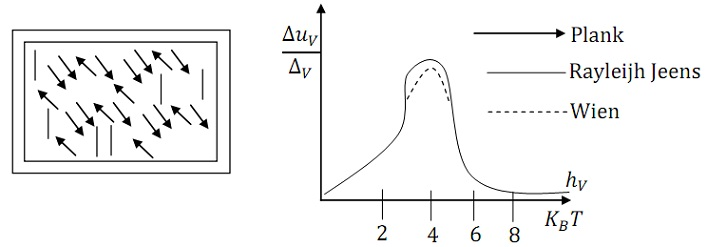1) The electromagnetic radiation within an oven is treated as a photon gas in the equilibrium by the oven walls.

2) Spectral distribution of the energy in black body radiation.

Lord Rayleigh studied the problem by using ideas of the classical physics and obtained an expression for UV dV Jeans introduced a numerical error in his formula and afterward corrected it. This so called Rayleigh Jean Law is of the form:

UV dV = (8πv2/C3) dvε

Here 'ε' is the mean energy of the oscillator, Lord Rayleigh and Sir James Jean employed the law of equipartition of energy and used ε = KBT by employing the result in the above equation, we get:

UV dV = (8πv2/C3) KBT dv

Ideal Bose-Einstein Gas:

We should first derive the Bose-Einstein distribution law and this will pave the way for Bose's derivation of Planck's law. If Planck was not convinced of the physical basis of his derivation, Bose introduced the correct process for treating a system on the basis of quantum statistics. Einstein expanded his ideas to the case of materials particles obeying the Bose statistics.

Throughout his investigations, Einstein came to the remarkable conclusion that Bose Einstein Gas can tend to a highly ordered state. This phenomenon, termed as Bose-Einstein condensation, was invoked by F. London to illustrate the super fluidity exhibited through liquid 4He.

Bose-Einstein Distribution Function:

Assume a system of 'N' non-interacting bosons occupying a volume 'V' and sharing a given energy 'U' levels of the system are very closely spaced.

In the limit of large 'V', the energy levels of the system are very closely spaced.

Therefore, we can bracket the energy levels into groups that might be called the energy cells.

This is termed as schematically in the figure shown below.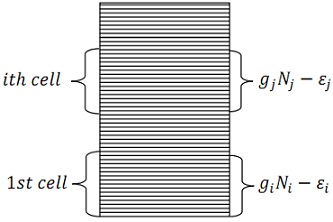(Energy level of the system bracketed into cells).

We suppose, devoid of any loss of generality, that the number of levels in the ith cell, gi, is very much greater than one (gi >> 1).

Whenever we treat energy as a continuous variable, the number of particles having energy 'ε' is represented by:

N(ε)/g(ε) = 1/[eβ(ε-μ) -1] = 1/(A-1 eβε -1)

This is termed as the Bose-Einstein distribution.

Bose Derivation of Planck' Law:

S.N Bose and Indian Physicist provide a very elegant derivation of Planck's law in the year 1924. He communicated his work to Einstein, who instantly acknowledged its importance.

He converted its findings into German language, got it published and his paper marks the birth of quantum statistics.

We take the equilibrium properties of electromagnetic radiation enclosed in the cavity of volume 'V' at temperature 'T'. You must recall that the distribution of energy among the different frequencies is independent of the nature of the walls of the container; it is a function of 'T' and 'V' only. We now want to find out the form of this function. From a quantum mechanical point of view, the radiation in the cavity can be considered as the collection of photons of various frequencies moving by the speed of light completely randomly. The photons of the similar frequency are indistinguishable.

This is an ideal illustration of a system of non-interacting, indistinguishable particles.

The energy of a photon of frequency 'v' is taken to be 'hv'. We must as well keep in mind that photons are particles having zero rest mass and spin (h/2π). Each and every photon can encompass two types of polarization. There are the two transverse modes that have no longitudinal photons.

In another words, the propagation vector and the polarization vector (providing the direction of polarization of the electric field related with the photon) are normal to one other. (This is an effect of the transversality of the electric field, i.e. V. E = 0)

You would as well appreciate the fact that the atoms can absorb or emit photons and the total number of photons is not constant.

In another words, we have only one constant, namely U = constant. This necessarily signifies that in equation written below require only one langrage multiplier β and α = 0 or A = 1.

N(ε)/g(ε) = 1/[eβ(ε-μ) -1] = 1/(A-1 eβε -1)

The above equation transforms to:

NV/gV = 1/(eβhv -1)

Assume that gV dV represent the number of quantum states between v and v + dv. We can derive an expression for this by employing the principles of quantum mechanics. Though, a simple argument can be employed to get the outcome.

UV dV = (EVdV/V) = (8πh/C3) [(V3 dv)/{exp(hv/KBT) -1}]

This is significant to note that Planck had derived the law via combining classical electromagnetic theory and the quantum hypothesis. On the other hand, Bose in a manuscript to Einstein in the year 1924 treated electromagnetic radiation as a system of indistinguishable particles which consists of the similar properties as particles of light which we now call photons. Following investigations led Einstein to the concept of stimulated emission that culminated in the growth of masers and lasers devices finding use in industry, medicine, energy production in fusion reactors and military application.

Liquid 4He and Bose-Einstein Condensation:

Hydrogen, the very first element in the periodic table, has contributed in a big measure to the growth of latest concepts and theories in physics. The second element, Helium is still more remarkable due to its existence from the sum that was discovered throughout a solar ellipse in India in the year 1868. Helium derives its name from the Greek word Helios that means the sun. Among all the elements, helium consists of the unique distinction of not solidifying even at the lowest attainable temperatures.

This is due to very weak forces between the helium atoms. (Its solid state can be obtained only beneath an external pressure of around 25 atmospheres). The P-T diagram, represented in the below points out the absence of a triple point. At atmospheric pressure, helium condenses to a normal liquid at 4.2 k. As the temperature is lowered further, liquid helium shows the other phase transition at 2.18 k.

You might anticipate helium to solidify. Rather it changes to the other liquid very surprising, in fact unique properties. The new state is termed as liquid He II to differentiate it from the phase above 2.18 k, which is termed liquid He I. You might remember that helium transition is a second order phase transition. The point at which the phase transition takes place is termed as the 'λ' point. This nomenclature is used because the shape of heat capacity curve looks like the Greek letter 'Lambda'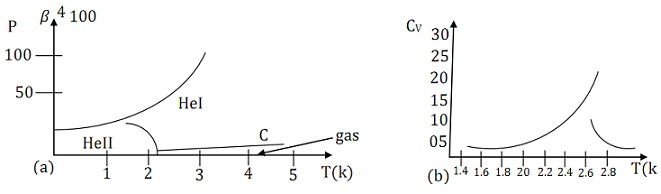A more dramatic manifestation of the unusual properties of liquid He II is its capability to flow via very narrow channels having zero viscosity. This property is termed as super fluidity.

A sequence of beautiful experiments has been designed to describe the effects of this property. Here, we should illustrate only one of them, viz, the fountain effect.

We take a U-tube and immerse it in a bath of liquid He II. The lower part of the tube is filled by emery powder. On shining a beam of light on the powder heat is absorbed and the super fluid tends to flow from the bath to the hotter area.

The motion is so violent that the jet of helium is forced up via the vertical tube and emerges as the fountain going as high as 30cm.

F. London (1938) recommended that the λ-transition must be recognized by Bose Einstein condensation. Einstein introduced a simple model that let us to apply BE statistics to liquid helium in order to gain insight to its peculiar behavior. Following him, we suppose that the distribution of excited states accessible to the atoms of liquid helium is that of a quantum gas and treat the ground state separately. If there are 'N' atoms in all, let Ng be in the ground state and Nex in the excited state.

Then, N = Ng + Nex -----

Tc = (h2/2πmKB)[N/2.612v]2/3

Tc is termed as the Bose Einstein condensation temperature.

Tutorsglobe: A way to secure high grade in your curriculum (Online Tutoring)